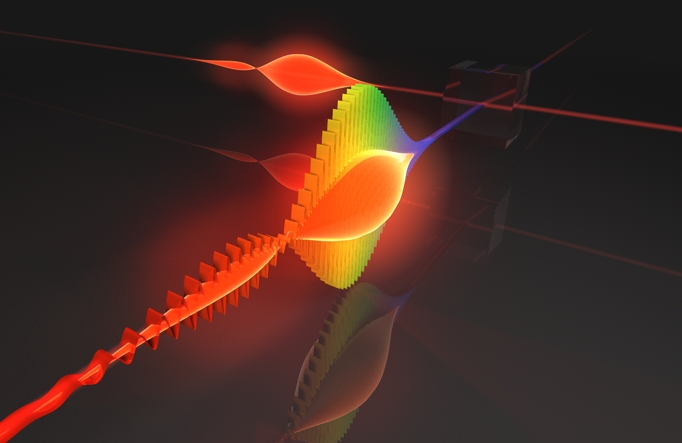# How well do you know electricity and magnetism ?Suppose that you live in some very, very weird universe where the speed of light is infinity. Now lets observe a infinitely long wire with current $I=10A$ passing trough it. Find the Magnetic field on a distance $r=5\text{ m}$ from the wire.

×